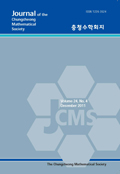상세검색
최근 검색어 전체 삭제
다국어입력
즐겨찾기0KCI등재 학술저널

# On minimal surfaces with Gaussian curvature analogous to Bianchi surfaces

• 등재여부 : KCI등재
• 2021.11
• 379 - 385 (7 pages)
• 9

We consider the local uniqueness of a catenoid under the condition for the Gaussian curvature analogous to Bianchi surfaces. More precisely, if a nonplanar minimal surface in R^3 has the Gaussian curvature K=-1/(U(u)+V(v))^2 for any functions U(u) and V(v) with respect to a line of curvature coordinate system (u,v), then it is part of a catenoid. To do this, we use the relation between a conformal line of curvature coordinate system and a Chebyshev coordinate system.

1. Introduction

2. Uniqueness of a catenoid

References